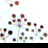# Subtracting attributes of different records based on common attribute

272
2
06-25-2014 09:22 AMNew Contributor II
I'm not sure if this is the right place to put this question.  If not, please let me know.

I am trying to find the angle between adjacent lines in a single layer.  I plan to accomplish this by subtracting the incidence angle of one line from that of its adjacent line. The lines come from polylines split at vertices, so the start coordinates of one line exactly match the end coordinates of another line.

I have a field with the incidence angle for each line, a field with the begin xy of the line, and a field with the end xy of the line.  I am trying to figure out how to have a script loop through the table, find the line whose beginningxy matches the endxy of the current line, and subtract the angles.  I am not very familiar with Python so I would greatly appreciate guidance.

Thanks!
Tags (2)
2 RepliesRegular Contributor II
If you split the lines using SplitLine (or the toolbox tool Split Line At Vertices)
The new lines will still have any original line ID you may have used
and be in order in the table from original from onwards.

You can identify the individual parts in order and add a segment ID.

Use an update cursor to run through the table and for each new origLineID,
you start with a segID of 1, and each time the origLineID stays the same, you increment the segID.

With line and segment ids, you can create a dictionary of a list of
incidence angles, in segment order, for each original line

Then it is always differences between adjacent pairs of angles.

This is all just off the cuff: no warranties...Occasional Contributor
This solution is assuming you have a multipart feature ... so a new row in your attribute table for each polyline segment.

What I'm doing here is cycling through the line geometries, getting the start and end point of each segment, and getting the azimuthal of the starting point.  (Note that if you have a longer line, your end azimuthal may be different due to it's great circle)

`import mathdef getBearing(part):    # Returns the initial bearing in degrees from north following the great    # circle path to the destination coordinates.    firstpoint = part.next()    lastpoint = part.next()    lat1 = math.radians(float(firstpoint.Y))    lat2 = math.radians(float(lastpoint.Y))    dLon = math.radians(float(lastpoint.X)-float(firstpoint.X))    y = math.sin(dLon) * math.cos(lat2)    x = math.cos(lat1) * math.sin(lat2) - \        math.sin(lat1) * math.cos(lat2) * math.cos(dLon)    brng = math.atan2(y, x)    return (math.degrees(brng) + 360) % 360;arcpy.AddField_management(YourFeatureClass,"angle","FLOAT")with arcpy.da.UpdateCursor(YourFeatureClass,["SHAPE@","angle"]) as cursor:    for row in cursor:        geom = row        part = geom.getPart(0)        bearing = getBearing(part)        print "Updating segment with bearing %f" % bearing        row = bearing        cursor.updateRow(row) `

This will update each row with it's azimuthal, then you can take it from there to calculate the differences between angles if needed.